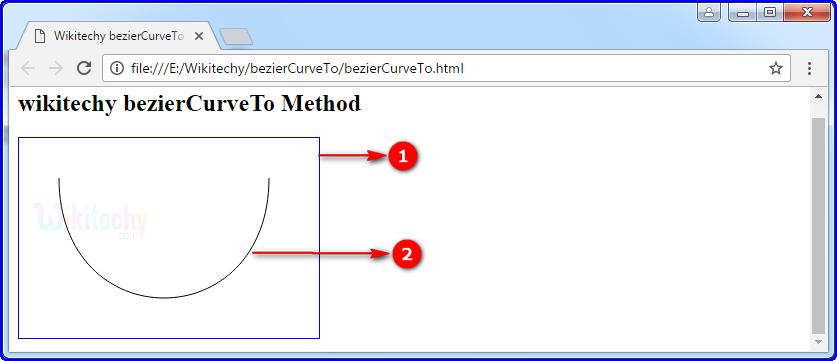# html tutorial - bezierCurveTo Method in HTML5 Canvas - html5 - html code - html formLearn html - html tutorial - Beziercurveto method in html5 canvas - html examples - html programs

• The bezierCurveTo() is a method of HTML canvas.
• A Bezier curve is a parametric curve.
• The bezierCurveTo() method adds a point to the current path by using the specified control points that represent a cubic Bezier curve.

## Parameter Values for bezierCurveTo() method in HTML5 Canvas:

parameter Description
cp1x The x-coordinate of the first Bezier control point.
cp1y The y-coordinate of the first Bezier control point.
cp2x The x-coordinate of the second Bezier control point.
cp2y The y-coordinate of the second Bezier control point.
x The x-coordinate of the ending point.
y The x-coordinate of the ending point.

## Code Explanation for bezierCurveTo() method in HTML5 Canvas:1. “wikitechyCanvas” is declared the id value of the HTML canvas.
2. The getElementById(); method is used to get the element with the specific id (“wikitechycanvas”).
3. The beginPath() method begins a path for Bezier curve.
4. The moveTo() method is used to move the path to the point(40,40) in the canvas.
5. The beziercurveTo() requires three points. The first two points are control points (40,200) (250,200) that are used in the cubic Bezier calculation and the last point (250,40) is the ending point for the curve. The starting point for the curve is the last point in the current path.
6. The stroke() method to actually draw the path on the canvas.

## Output for bezierCurveTo() method in HTML5 Canvas:1. The HTML rectangle canvas with blue color border.
2. The Bezier curve is displayed on HTML canvas.

## Output Coordinate Explanation for bezierCurveTo() method in HTML5 Canvas:1. Start point(40,40)
2. Control point 1(40,200)
3. Control point 2(250,200)
4. End point (250,40)

## Browser Support for bezierCurveTo() method in HTML5 Canvas:Yes 9.0 Yes Yes Yes